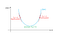# Stanford/Coursera Machine Learning: Linear Regression, Gradient Descent

Problem: Given a dataset of Housing Prices in Portland Oregon, create a model that can help you set a price for your house.

Notation for the course

m: Number of training examples
x: ‘Input’ variables/features
y: ‘Output’ variable/’target’ variable
(x, y): One training example
iᵗʰ training example (iᵗʰ row in the table): (x⁽ⁱ⁾, y⁽ⁱ⁾)

# Linear Regression

Linear regression is used for finding linear relationship between a target (or dependent variable) and one or more explanatory variables (predicting parameters). The case of one explanatory variable is called simple linear regression. For more than one explanatory variable, the process is called multiple linear regression.

## Supervised learning: Flow of implementation

In case of multiple features as in Figure 3 (Living Area, Number of Bedrooms): iᵗʰ training example: (x₁⁽ⁱ⁾, x₂⁽ⁱ⁾, x₃⁽ⁱ⁾, .. xₙ⁽ⁱ⁾, y⁽ⁱ⁾), where xₙ is the nth feature in the dataset.

In the above example, x₁ denotes value for Living Area feature and x₂ for Number of Bedrooms feature.

h(x) = θ₀ + θ₁x₁ + θ₂x₂

h(x) is the price the hypothesis predicts for house with feature x (x₁, x₂)

For conciseness, define x₀ = 1,

n: number of features

• θᵢ’s are called the parameters of the learning algorithm and θᵢ∈ℝ.
• It is the job of the learning algorithm to use the training set to learn appropriate parameters θ.

How do we choose parameters θ so that our hypothesis h will make accurate predictions about housing prices?

• We can run our hypothesis on our training dataset and predict the prices of houses in that set.
• Here we can have our primary goal as minimising the square-difference between the predicted housing price and the actual housing price. ie compute the value of parameters θ for which the said difference is minimum.
• Here J(θ) is the cost function which is required to be minimised over. We’ll talk about couple of different algorithms (Gradient descent in this post and Normal Equations in another) to minimize the cost function J(θ).

• Keep changing θ to reduce J(θ), until we reach to the minima for J(θ).

Mapping J(θ)

On the y-axis, there is the cost J(θ) against parameters θ1 and θ2 on x-axis and z-axis respectively.

We can consider the graph as a mountain and us as being at the top of it. What step or set of steps should we take so that our altitude decreases? Or rather in which direction should we move? The Gradient Descent algorithm works in the similar way.

Gradient descent is based on the observation that if the multi-variable function F(x) is defined and differentiable in a neighborhood of a point a, then F(x) decreases fastest if one goes from a in the direction of the negative gradient of F at a, -∇ F(a).Figure 6: Move in the direction opposite to the slope | Source Towards Data Science

If the function is non-convex, the initial starting point(θ₀ and θ₁) may decide if you get to the global minima or get stuck at a local one.

• In this method we need to sum over all training examples for each steps.
• Batch Gradient Descent is not a good algorithmic idea for huge datasets

I. In case of one training example

After taking derivatives (finding slope),

(Learning rule) Update:

α is the parameter of the algorithm called the Learning Rate. It controls how large a step you take.

II. For m training examples,

Repeat till convergence:

The above is an example of Batch Gradient Descent, ie on every step of gradient descent we are going through entire dataset. In stochastic gradient descent you instead take a sample while computing the gradient.

• Here we use one training example at each iteration instead of using whole dataset to sum all for every steps.
• SGDA is used for larger dataset trainings.
• SGDA tends to wander around the global minima and won’t actually converge to it exactly.

For Ordinary Least Squares, Function J(θ) is a quadratic one and hence would have a bow shape, having 1 global minima.

Written by

## More From Medium

### Implementing Linear Regression in Python and Ruby

#### 83

Welcome to a place where words matter. On Medium, smart voices and original ideas take center stage - with no ads in sight. Watch
Follow all the topics you care about, and we’ll deliver the best stories for you to your homepage and inbox. Explore
Get unlimited access to the best stories on Medium — and support writers while you’re at it. Just \$5/month. Upgrade